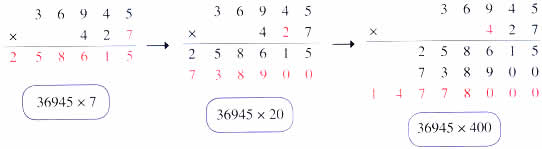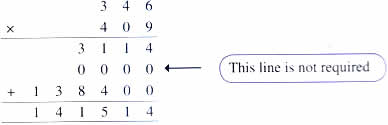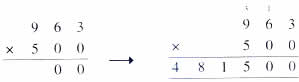Thursday , July 7 2022# NCERT 5th Class (CBSE) Mathematics: The Four Operations And Their Application

## MULTIPLICATION

### Properties of Multiplication:

These are some properties of multiplication that you are familiar with.

1. Any number multiplied by 0 gives zero as the product.
2. Any number multiplied by 1 gives the same number as the product.
3. When the order of the factors is changed the product does not change.

### Multiplying by a 3-Digit Number### Multiplying by Numbers with 0(b)## चुनौती हिमालय की 5th NCERT CBSE Hindi Rimjhim Ch 18

चुनौती हिमालय की 5th Class NCERT CBSE Hindi Book Rimjhim Chapter 18 प्रश्न: लद्दाख जम्मू-कश्मीर राज्य में …